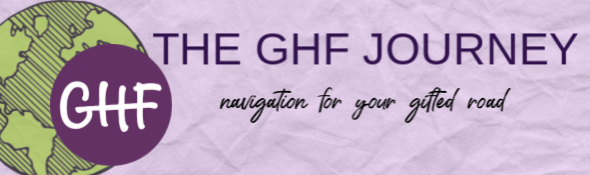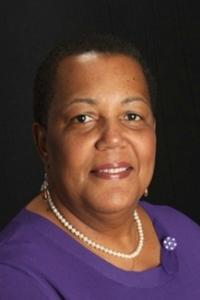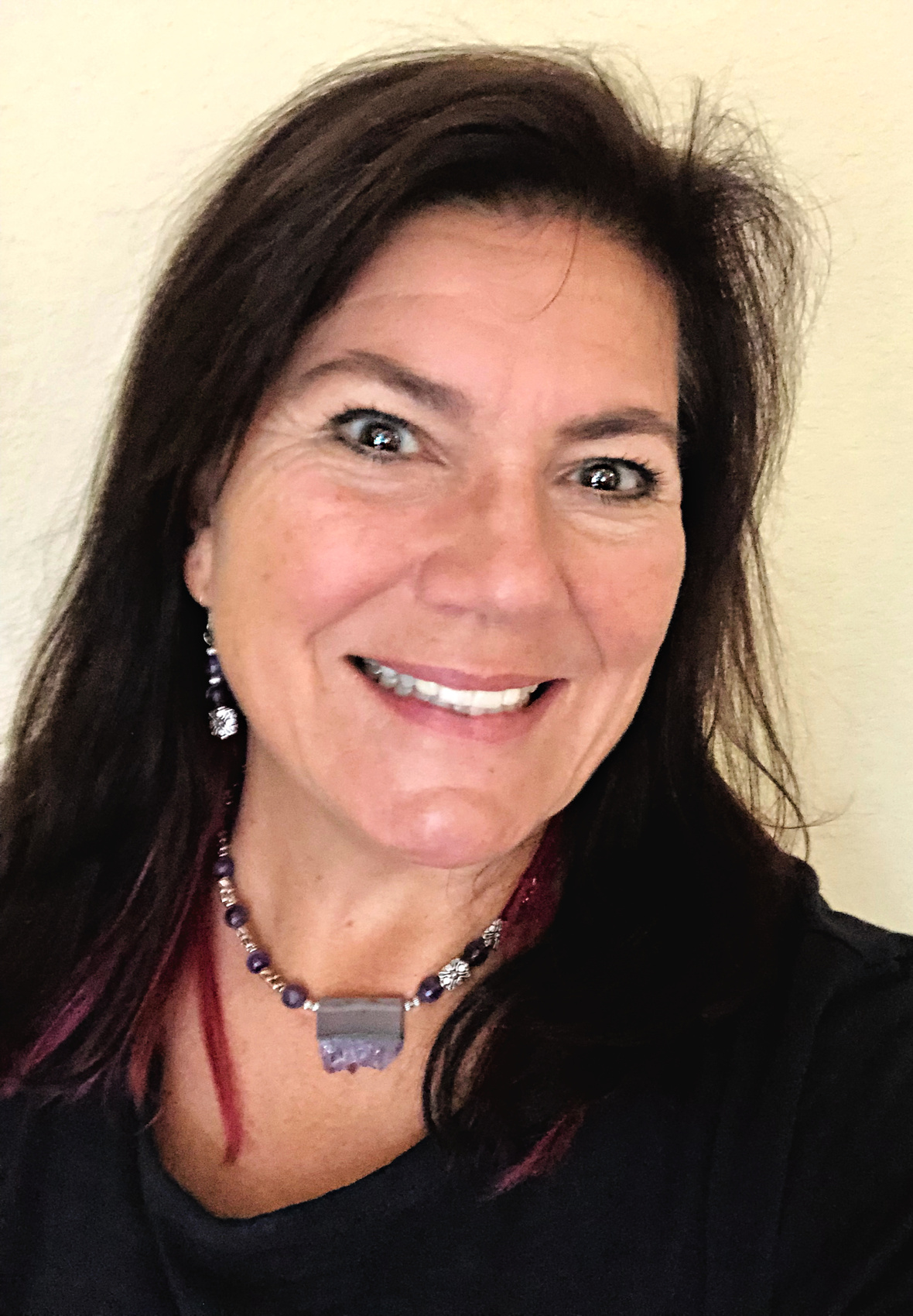Like   Tweettable.module-2{width:53.77%;padding:0}table div table+table+table div table{width:53.77%;float:none;margin-left:auto;margin-right:auto;padding:0}table div table+table+table div table a{border:0 none;text-decoration:none}table div table+table+table div table img{width:100%!important;border:0 none;text-decoration:none}table div table+table+table div table td{width:100%;padding:0}/* styles */

# Top 10 Advocacy Tips for Parents of Black & Hispanic Gifted Students

School districts nationwide are being challenged by scholars, families, and educators to do a better job of including more students of culturally diverse backgrounds in gifted education services. Your role as an advocate for your child is critical. School districts will become more responsive when parents speak up and stand up for their children’s rights.

If you believe your child/teen has high potential/ is gifted as demonstrated by their unique responses to the world around them, school performance, exceptional gifts as demonstrated in community or church related events, arts activities, test scores or school grades and they have NOT been considered for the school district advanced learner or gifted education program services, use the following ten advocacy strategies to help get your child noticed, identified, and served in gifted education or advanced classes.

Read the rest of Dr. Davis's article here...

## Joy Lawson Davis, Ed.Dtable div table+table+table+table+table+table+table div table{width:100%;padding:0}table div table+table+table+table+table+table+table div table img{width:96.23%;padding:0;float:none}table div table+table+table+table+table+table+table div table td{width:100%;padding:0 1.88% 18px}/* styles */table.module-7{width:22.64%;padding:0}table div table+table+table+table+table+table+table+table div table{width:22.64%;float:none;margin-left:auto;margin-right:auto;padding:0}table div table+table+table+table+table+table+table+table div table a{border:0 none;text-decoration:none}table div table+table+table+table+table+table+table+table div table img{width:100%!important;border:0 none;text-decoration:none}table div table+table+table+table+table+table+table+table div table td{width:100%;padding:0}/* styles */
 table div table+table+table+table+table+table+table+table+table div table{width:100%;padding:0}table div table+table+table+table+table+table+table+table+table div table img{width:96.23%;padding:0;float:none}table div table+table+table+table+table+table+table+table+table div table td{width:100%;padding:0 1.88% 18px}/* styles */## From the EditorCeli Trépanier

Navigating your gifted road can be rife with potholes, bumps, blind curves, and sometimes seemingly insurmountable roadblocks. Whether helping your child navigate through a world which often stereotypes our gifted children, or finding your way as a gifted adult with unique social and emotional needs, we could all use a little understanding of who we gifted people are.

The only way to create a better world for gifted people everywhere, no matter their zip code, the color of their skin, or the diversity or learning differences they possess, is through advocacy.

Through this issue of the GHF Journey, it is my fervent and heartfelt hope that you will be inspired to raise your voice in advocacy for the gifted. One voice leads to many.

It matters not how you choose to advocate. We all spread awareness in our own way—all effective, all necessary. It only matters that you choose to.

Whether in big ways or small ways, every word that spreads awareness and a better understanding of giftedness benefits us all. Please lend your voice.

Celi Trépanier
Editorial Director for GHF Learners

 table div table+table+table+table+table+table+table+table+table+table+table+table div table{width:100%;padding:0}table div table+table+table+table+table+table+table+table+table+table+table+table div table img{width:96.23%;padding:0;float:none}table div table+table+table+table+table+table+table+table+table+table+table+table div table td{width:100%;padding:0 1.88% 18px}/* styles */table.module-12{width:91.51%;padding:0}table div table+table+table+table+table+table+table+table+table+table+table+table+table div table{width:91.51%;float:none;margin-left:auto;margin-right:auto;padding:0}table div table+table+table+table+table+table+table+table+table+table+table+table+table div table a{border:0 none;text-decoration:none}table div table+table+table+table+table+table+table+table+table+table+table+table+table div table img{width:100%!important;border:0 none;text-decoration:none}table div table+table+table+table+table+table+table+table+table+table+table+table+table div table td{width:100%;padding:0}/* styles */
 table div table+table+table+table+table+table+table+table+table+table+table+table+table+table div table{width:100%;padding:0}table div table+table+table+table+table+table+table+table+table+table+table+table+table+table div table img{width:96.23%;padding:0;float:none}table div table+table+table+table+table+table+table+table+table+table+table+table+table+table div table td{width:100%;padding:0 1.88% 18px}/* styles */table.module-14{width:20.75%;padding:0}table div table+table+table+table+table+table+table+table+table+table+table+table+table+table+table div table{width:20.75%;float:none;margin-left:auto;margin-right:auto;padding:0}table div table+table+table+table+table+table+table+table+table+table+table+table+table+table+table div table a{border:0 none;text-decoration:none}table div table+table+table+table+table+table+table+table+table+table+table+table+table+table+table div table img{width:100%!important;border:0 none;text-decoration:none}table div table+table+table+table+table+table+table+table+table+table+table+table+table+table+table div table td{width:100%;padding:0}/* styles */
 table div table+table+table+table+table+table+table+table+table+table+table+table+table+table+table+table div table{width:100%;padding:0}table div table+table+table+table+table+table+table+table+table+table+table+table+table+table+table+table div table img{width:96.23%;padding:0;float:none}table div table+table+table+table+table+table+table+table+table+table+table+table+table+table+table+table div table td{width:100%;padding:0 1.88% 18px}/* styles */# In the World of Gifted Advocacy, We All Have a Voice

 table.module-17{width:79.06%;padding:0}table div table+table+table+table+table+table+table+table+table+table+table+table+table+table+table+table+table+table div table{width:79.06%;float:none;margin-left:auto;margin-right:auto;padding:0}table div table+table+table+table+table+table+table+table+table+table+table+table+table+table+table+table+table+table div table a{border:0 none;text-decoration:none}table div table+table+table+table+table+table+table+table+table+table+table+table+table+table+table+table+table+table div table img{width:100%!important;border:0 none;text-decoration:none}table div table+table+table+table+table+table+table+table+table+table+table+table+table+table+table+table+table+table div table td{width:100%;padding:0}/* styles */

Gifted advocates throughout the world share one commonality: our goal is to see every gifted child receive the educational, social, and emotional support they need to fulfill their life's potential regardless of their zip code, the color of their skin, or any diversity or learning differences they possess. We want every gifted child to have the best opportunity to become who they were meant to be, whatever that may be.

Gifted advocates everywhere are tackling our mutual goal in various ways, using a multitude of tools and marching down different paths. This is as it should be—creating an understanding for the world's gifted people necessitates a far-reaching, multilevel approach.

 table div table+table+table+table+table+table+table+table+table+table+table+table+table+table+table+table+table+table+table+table div table{width:100%;padding:0}table div table+table+table+table+table+table+table+table+table+table+table+table+table+table+table+table+table+table+table+table div table img{width:96.23%;padding:0;float:none}table div table+table+table+table+table+table+table+table+table+table+table+table+table+table+table+table+table+table+table+table div table td{width:100%;padding:0 1.88% 18px}/* styles */table.module-20{width:22.64%;padding:0}table div table+table+table+table+table+table+table+table+table+table+table+table+table+table+table+table+table+table+table+table+table div table{width:22.64%;float:none;margin-left:auto;margin-right:auto;padding:0}table div table+table+table+table+table+table+table+table+table+table+table+table+table+table+table+table+table+table+table+table+table div table a{border:0 none;text-decoration:none}table div table+table+table+table+table+table+table+table+table+table+table+table+table+table+table+table+table+table+table+table+table div table img{width:100%!important;border:0 none;text-decoration:none}table div table+table+table+table+table+table+table+table+table+table+table+table+table+table+table+table+table+table+table+table+table div table td{width:100%;padding:0}/* styles */
 table div table+table+table+table+table+table+table+table+table+table+table+table+table+table+table+table+table+table+table+table+table+table div table{width:100%;padding:0}table div table+table+table+table+table+table+table+table+table+table+table+table+table+table+table+table+table+table+table+table+table+table div table img{width:96.23%;padding:0;float:none}table div table+table+table+table+table+table+table+table+table+table+table+table+table+table+table+table+table+table+table+table+table+table div table td{width:100%;padding:0 1.88% 18px}/* styles */# Fearless advocacy: A day in the life of a gifted child's parent

 table div table+table+table+table+table+table+table+table+table+table+table+table+table+table+table+table+table+table+table+table+table+table+table+table+table+table div table{width:100%;padding:0}table div table+table+table+table+table+table+table+table+table+table+table+table+table+table+table+table+table+table+table+table+table+table+table+table+table+table div table img{width:96.23%;padding:0;float:none}table div table+table+table+table+table+table+table+table+table+table+table+table+table+table+table+table+table+table+table+table+table+table+table+table+table+table div table td{width:100%;padding:0 1.88% 18px}/* styles */# For Gifted Kids And Their Teachers — Strategies For SuccessMaybe you are an enthusiastic, hungry learner. You have so many questions and so many answers; your drive to analyze and create is massive and never-ending. Your intense curiosity annoys your fellow students and rattles your teachers.
Was this your experience in first grade? Is this your story even now that you’re in grad school? If so, it can be deeply painful and frustrating. You may blame yourself for your too muchness and your seemingly inadequate communication and social skills. You may have been labeled a know-it-all but you wonder how that’s possible when you feel like a want-to-know-it-all and a slacker.

 table div table+table+table+table+table+table+table+table+table+table+table+table+table+table+table+table+table+table+table+table+table+table+table+table+table+table+table+table+table div table{width:100%;padding:0}table div table+table+table+table+table+table+table+table+table+table+table+table+table+table+table+table+table+table+table+table+table+table+table+table+table+table+table+table+table div table img{width:96.23%;padding:0;float:none}table div table+table+table+table+table+table+table+table+table+table+table+table+table+table+table+table+table+table+table+table+table+table+table+table+table+table+table+table+table div table td{width:100%;padding:0 1.88% 18px}/* styles */## The President's Club - Elite GHF Donors

 /* styles */ For those of you who can give at least \$500 we have created a special recognition program where you will be listed on the GHF website and in our monthly newsletter, The GHF Journey, as valued members of the community. Donations may be kept anonymous.

## Platinum Donor -- \$2,500

 ▪ Barry Gelston, Mr. Gelston's One Room Schoolhouse

## Silver Donors -- \$500

▪ Rosemary Guillette
▪ Dr. Melanie Hayes, Big Minds Unschool
▪ Heidi Molbak, Seed Starter Educational Consulting
▪ Dan Peters, Summit Center
▪ Magalie Pinney, State Street's Do More Grant
▪ Jade Ann Rivera, Sunnyside Micro-School